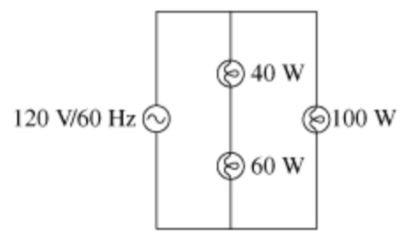# Problem: Light bulbs labeled 40 W, 60 W, and 100 W are connected to a 120 V/60 Hz power line as shown in the figure.What is the rate at which energy is dissipated in each bulb? Express your answers using two significant figures.

###### FREE Expert Solution

Power in circuits:

$\overline{){\mathbf{P}}{\mathbf{=}}{\mathbf{V}}{\mathbf{i}}{\mathbf{=}}{{\mathbf{i}}}^{{\mathbf{2}}}{\mathbf{R}}{\mathbf{=}}\frac{{\mathbf{V}}^{\mathbf{2}}}{\mathbf{R}}}$

Equivalent resistance for resistors in series:

$\overline{){{\mathbf{R}}}_{{\mathbf{eq}}}{\mathbf{=}}{{\mathbf{R}}}_{{\mathbf{1}}}{\mathbf{+}}{{\mathbf{R}}}_{{\mathbf{2}}}{\mathbf{+}}{\mathbf{.}}{\mathbf{.}}{\mathbf{.}}{\mathbf{+}}{{\mathbf{R}}}_{{\mathbf{n}}}}$

In this problem, we have two branches connected to 120V. One branch has two bulbs (40W and 60W) while the other branch has a 100W resistor.

86% (485 ratings)###### Problem Details

Light bulbs labeled 40 W, 60 W, and 100 W are connected to a 120 V/60 Hz power line as shown in the figure.What is the rate at which energy is dissipated in each bulb? Express your answers using two significant figures.